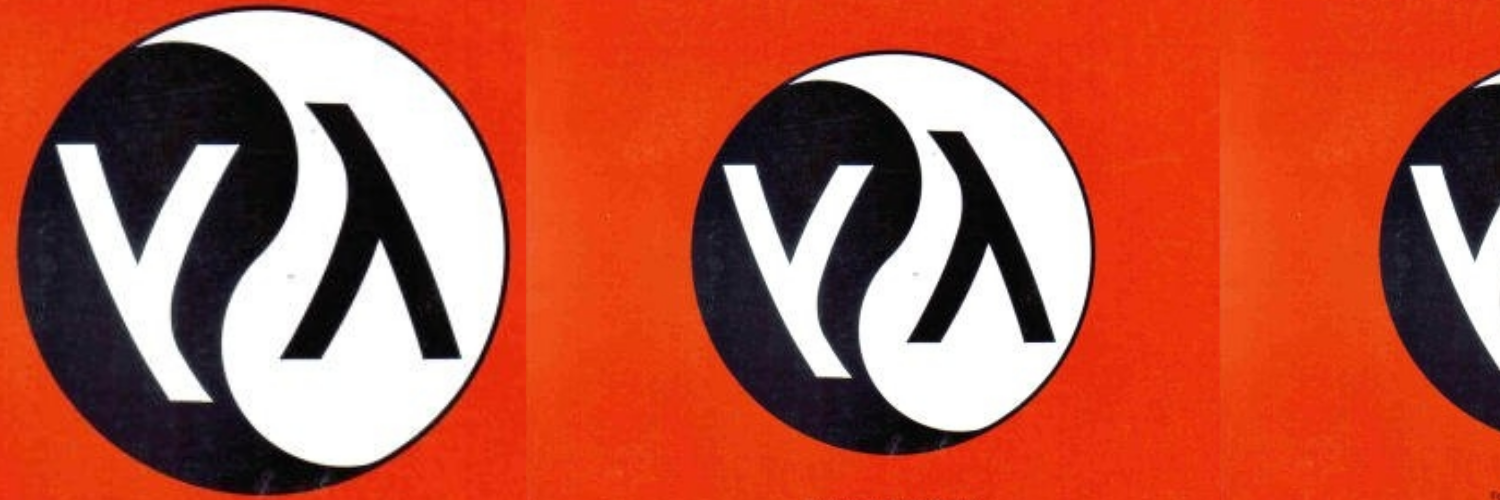# Functional Programming

## Separating Program Evaluation From Description

Stream, Lazy, Map, Filter, EVAL

5.3 Separating program evaluation from description This is the title of Chapter 5.3 from the book Functional Programming in Kotlin. Everyone knows that a program is a list of instructions that will be executed/evaluated in the order they are written in. fun exec() { doThis() doThat() doMore() } So the description decides the evaluation. What does it mean to separate them? I mean, can they be different?

## The Most Beautiful Program Ever Written

A Lisp interpreter written in LispIt’s my 5th time trying to follow this video and things finally start to click in my head. So I think if I write down what is talked in the video, then I can just read my blog post for another 15 times instead of going through the video, that would be convenient. OK, here is a gist of it. This guy is writing an Scheme interpreter in Scheme. Let’s start from setting up the environment first so we can type along afterwards.

## Tree Vs Iterative Fibbonacci Numbers

Two types of recursionBeing a programmer, you should be very familiar with Fibbonacci numbers. It is often being introduced when teaching recursion. Tree Recursion Most likely the implementation of a function that calculate fib number is as follows: (defn fib-tree [n] (cond (= n 0) 0 (= n 1) 1 :else (+ (fib-tree (- n 1)) (fib-tree (- n 2))))) This is just a direct translation from the Fibonacci number definition. Since it is straightforward and easy to understand, most textbooks use it as an typical example for illustrating recursive function.

## Understand Clojure Transducers 1